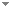Switch to: References

# Expanding Quasi-MV Algebras by a Quantum Operator

Studia Logica 87 (1):99-128 (2007)

 Quantum Computational Logic with Mixed States.Hector Freytes & Graciela Domenech - 2013 - Mathematical Logic Quarterly 59 (1-2):27-50.In this paper we solve the problem how to axiomatize a system of quantum computational gates known as the Poincaré irreversible quantum computational system. A Hilbert-style calculus is introduced obtaining a strong completeness theorem. Direct download (2 more)     Export citationBookmark1 citation Quantum Computational Structures: Categorical Equivalence for Square Root qMV -Algebras.Hector Freytes - 2010 - Studia Logica 95 (1-2):63 - 80.In this paper we investigate a categorical equivalence between square root qMV-algehras (a variety of algebras arising from quantum computation) and a category of preordered semigroups. Direct download (4 more)     Export citationBookmarkThe Lattice of Subvarieties of $${\sqrt{\prime}}$$ Quasi-MV Algebras.T. Kowalski, F. Paoli, R. Giuntini & A. Ledda - 2010 - Studia Logica 95 (1-2):37-61.In the present paper we continue the investigation of the lattice of subvarieties of the variety of ${\sqrt{\prime}}$ quasi-MV algebras, already started in . Beside some general results on the structure of such a lattice, the main contribution of this work is the solution of a long-standing open problem concerning these algebras: namely, we show that the variety generated by the standard disk algebra D r is not finitely based, and we provide an infinite equational basis for the same variety. Direct download (3 more)     Export citationBookmark1 citation